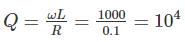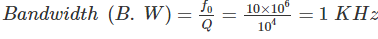Courses

# Electrical Circuits 2 MCQ

## 20 Questions MCQ Test Mock Test Series for SSC JE Electrical Engineering (Hindi) | Electrical Circuits 2 MCQ

Description
This mock test of Electrical Circuits 2 MCQ for Electrical Engineering (EE) helps you for every Electrical Engineering (EE) entrance exam. This contains 20 Multiple Choice Questions for Electrical Engineering (EE) Electrical Circuits 2 MCQ (mcq) to study with solutions a complete question bank. The solved questions answers in this Electrical Circuits 2 MCQ quiz give you a good mix of easy questions and tough questions. Electrical Engineering (EE) students definitely take this Electrical Circuits 2 MCQ exercise for a better result in the exam. You can find other Electrical Circuits 2 MCQ extra questions, long questions & short questions for Electrical Engineering (EE) on EduRev as well by searching above.
QUESTION: 1

### If the energy is supplied form a source, whose resistance is 1 Ohm, to a load of 100 Ohms the source will be

Solution:

The internal resistance of an ideal voltage source should be zero and practically it is very low.

Given that, source resistance is 1 ohm

Hence the source will be a voltage source

QUESTION: 2

### A lamp rated at 60V, 40W is to be connected across 230V. What is the value of resistance to be connected in series with lamp?

Solution:

Rating of lamp = 60V, 40 W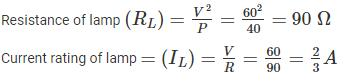When lamp is connected, maximum current that can be flow through the circuit is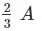Let RS be the series resistance.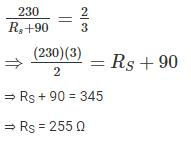QUESTION: 3

### The sum of currents entering a junction is 9 A. If the current leaves the junction form 3 different paths having the same resistance, the current leaving from any one of the path will be:

Solution:

By KCL,

The sum of current entering a junction is equal to the sum of current leaving at that junction.

⇒ Sum of currents leaving = 9 A

This 9 A current will flow through three resistances having same value.

The current will be some through each resistor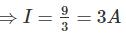QUESTION: 4

A voltage source and two resistors are connected in parallel. Suppose that Vs = 150V, R= 50Ω and R2 = 25Ω. Then each resistance contain current?

Solution: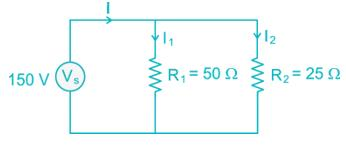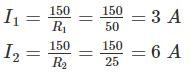QUESTION: 5

A current source and a voltage source are connected in series with a resistor as shown below. Suppose that Vs = 10V, is = 3A and R = 5Ω. What is the voltage across the resistor and the power absorbed by the resistor?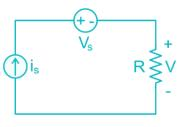Solution: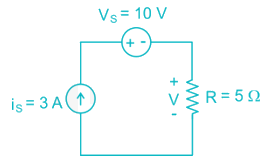QUESTION: 6

The voltage source in the given circuit supplies 24W of power. The current source supplies 6.0 W. Determine the value of the resistance R and R2: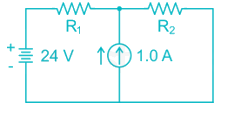Solution: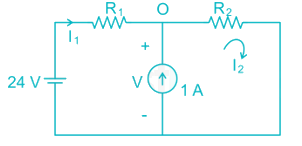Given that, voltage source supplies 24 W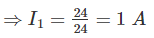By applying KVL,

- 24 + I1 R1 + I2 R2 = 0

⇒ I2 R2 = 24 – R1 → (1)

Given that, current source supplies 6 W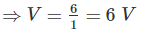By KVL in second loop

I2 R2 = 6 → (2)

From (1) and (2)

⇒ R1 = 18 Ω

By KCL at point ‘O’

⇒ I2 = 1 + I1 = 2 A

We know that, I2 R2 = 6

⇒ R2 = 3 Ω

QUESTION: 7

The Thevenin equivalent resistance (RTh) for the circuit shown in fig. is: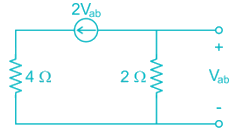Solution: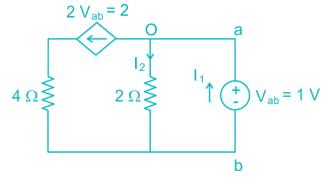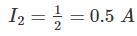By KCL at point ‘O’

I1 = I2 + 2 = 0.5 + 2 = 2.5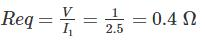QUESTION: 8

Applying Norton’s Theorem, the Norton’s equivalent circuit to the left of the terminals “a” and “b” in the below circuit is having equivalent current source (IN) and equivalent resistance (RN)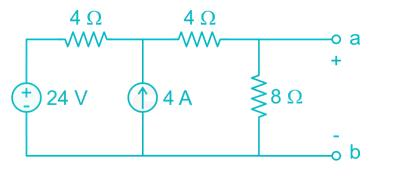Solution:

To find Norton’s resistance, we need to short circuit the voltage source and open circuit the current source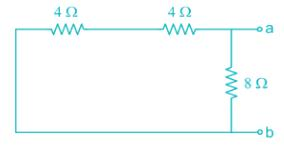RN = (4 + 4) || 8 = 4 Ω

To find the Norton’s current, we need to find short circuit current across terminals a-b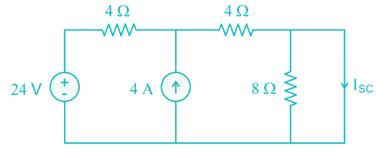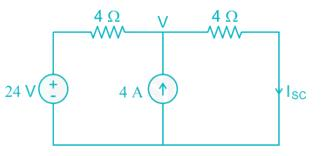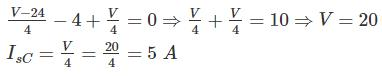QUESTION: 9

Find the value of Req in the figure given below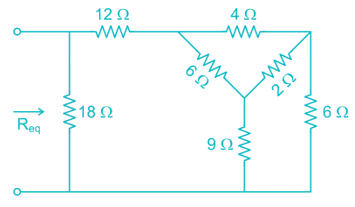Solution: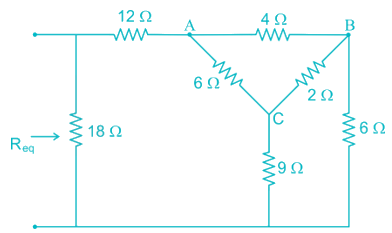By converting Delta (ABC) in to star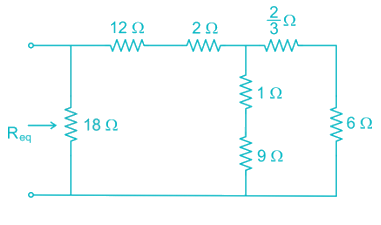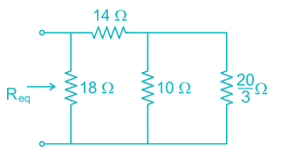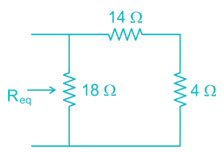Req = 18 || (14 + 4) = 9 Ω

QUESTION: 10

Consider the following circuit and find the current through the 6Ω resistor.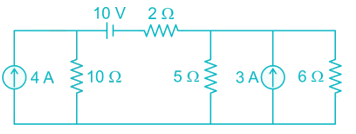Solution:

Let the current passing through 6 Ω resistor is I.

By source transformation,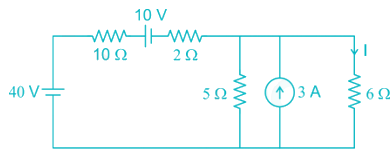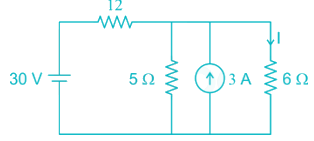By source transformation,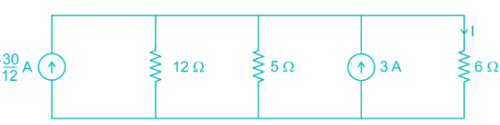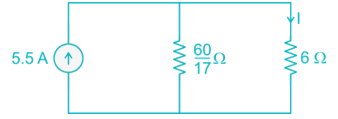By current division,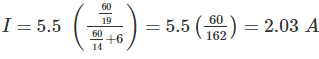QUESTION: 11

The Norton current at terminals a and b of the circuit shown at Figure: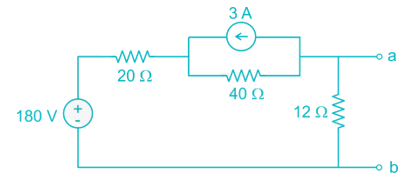Solution:

To find Norton’s current, we need to find the short circuit current across the terminals a and b.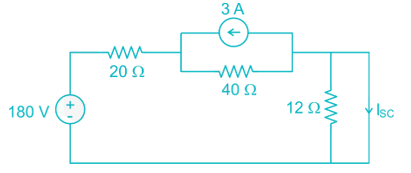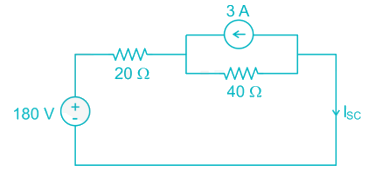By source transformation,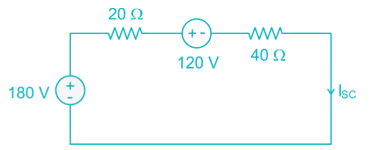By KVL,

-180 + 20 ISC + 120 +40 ISC = 0

⇒ ISC = 1 A

QUESTION: 12

Given condition justifies which network theorems- the load impedance should be complex conjugate of the internal impedance of the active network.

Solution:

According to maximum power transfer theorem, in AC circuits the load impedance should be complex conjugate of the internal impedance of the active network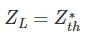QUESTION: 13

Three capacitors of 10μF, 20μF and 40μF are connected in parallel across 100 V. The total charge stored in capacitors is:

Solution: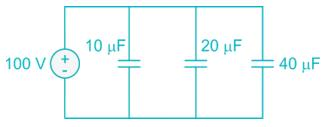Ceq = 10 + 20 + 40 = 70 μF

Charge, Q = CV = (70 μ F) (100 V) = 7 × 10-3 C

QUESTION: 14

An ac voltage is described by v(t) = 10 cos (400 πt), find out frequency and RMS value of voltage.

Solution:

V(t) = 10 cos (400 π t)

ω = 400 π

⇒ 2 π f = 400 π

⇒ f = 200 Hz

Vm = 10 V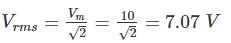QUESTION: 15

An inductive coil of 0.2 H is connected to 200 V, 50 Hz source. What are the inductive reactance and RMS current in the circuit?

Solution:

Given that, inductance = 0.2 H

Voltage = 200 V

Inductive reactance = 2 π fL = 2 π × 50 × 0.2 = 62.8 Ω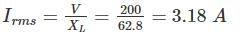QUESTION: 16

A series resonant circuit has R = 2Ω, L = 1mH and C = 0.1 μF, the value of quality factor Q is:

Solution:

Given that, R = 2 Ω, L = 1 mH, C = 0.1 μ F

For series resonant circuit,QUESTION: 17

A ramp voltage V(t) = 100V is applied to an RC differentiating circuit with R = 5kΩ and C = 4μF. The maximum output voltage is

Solution: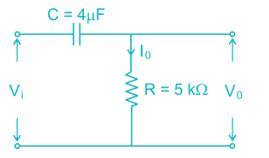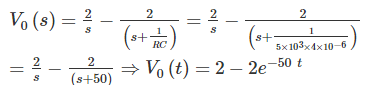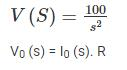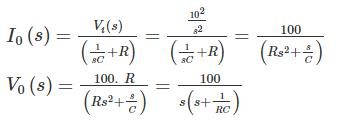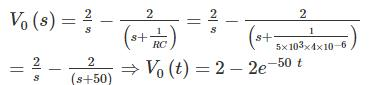Maximum value of V(t) = 2 V

QUESTION: 18

In an a.c. circuit, v = 50sin(ωt+60∘),i = 10cos(ωt)then the power factor of a.c. circuit will be:

Solution:

Given that, V = 50 sin (ω t + 60°)

i = 10 cos (ω t)

i = 10 cos (ω t)

i = 10 sin (ω t + 90°)

Phase difference (ϕ) = 30°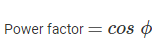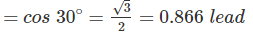QUESTION: 19

A balanced star connected load of 8 + j6 ohms per phase is connected to a 3-phase 230V supply. Power being consumed by the load is.

Solution:

Z = 8 +j 6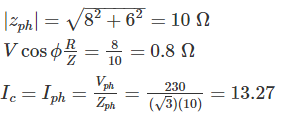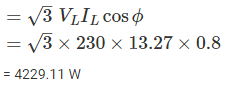= 4229.11 W

QUESTION: 20

What will be the bandwidth of a series resonant circuit provided it has an inductive reactance of 1000 Ohm, a capacitive reactance of 1000 Ohm a resistance of 0.1 Ohm? It also know that the resonant frequency is 10MHz.

Solution:

Given that, XL = 1000 Ω

XC = 1000 Ω

R = 0.1 Ω

F0 = 10 MHz